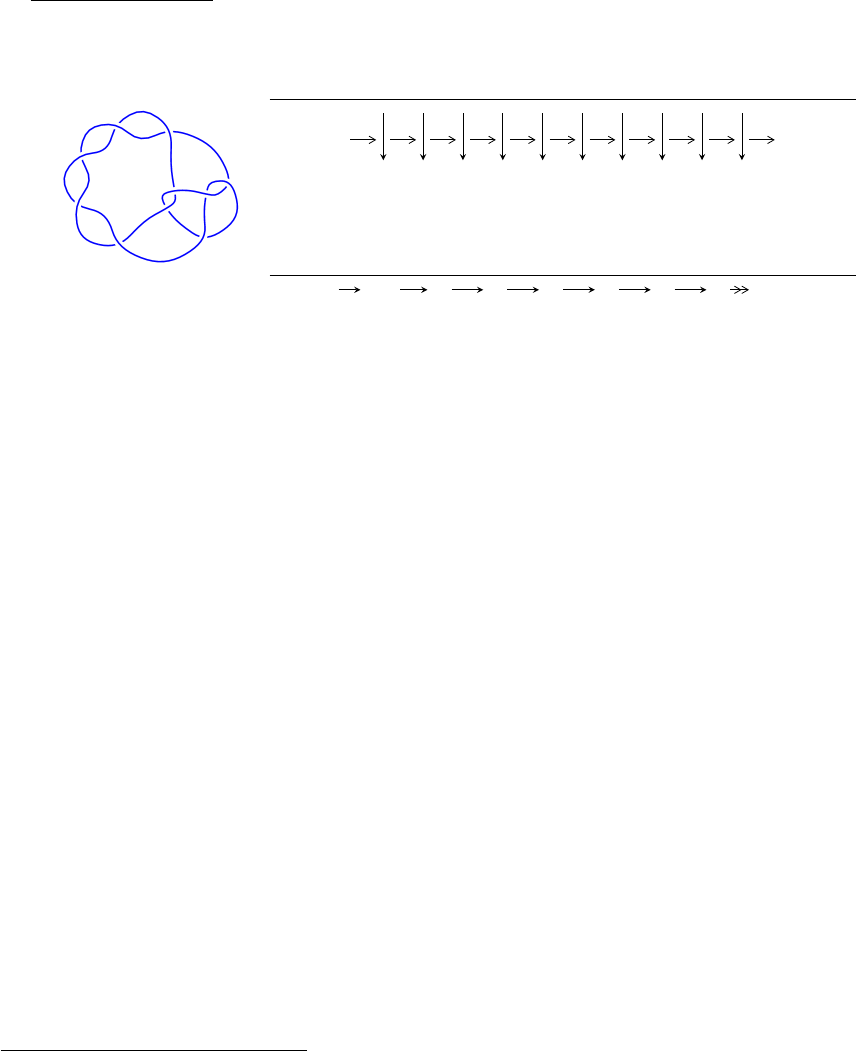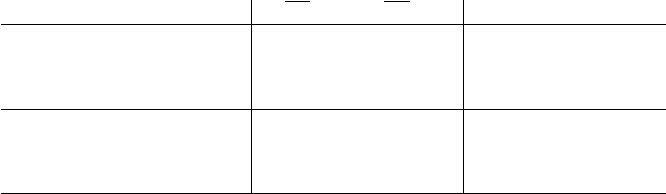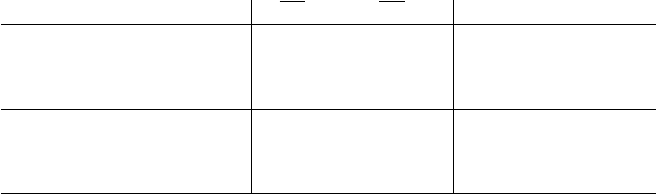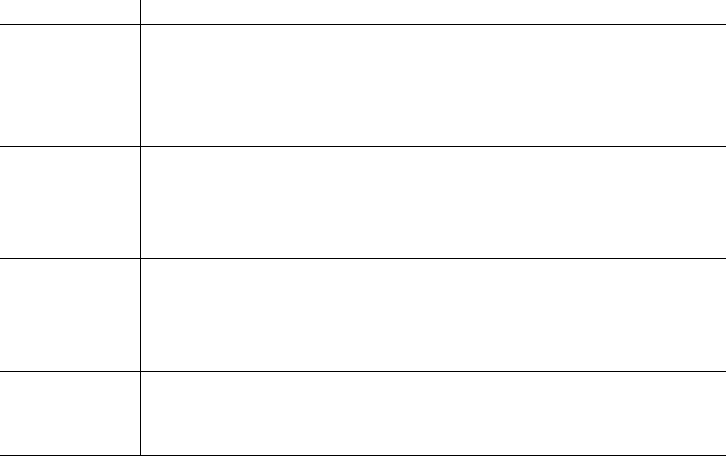10
124
(K10n
21
)
1
Arc Sequences
5 6 7 10 2 3 10 7 4 8
Solving Sequence
7,10 4,8
3 6 2 5 1 9
c
7
c
3
c
6
c
2
c
5
c
1
c
9
c
4
, c
8
, c
10
Representation Ideals
I =
2
\
i=1
I
u
i
I
u
1
= hu
2
3u + 1, a u + 1, b + 4u 2i
I
u
2
= hu
2
+ u 1, b, a + u + 1i
There are 2 irreducible components with 4 representations.
1
The knot diagram image is adapter from “C. Livingston and A. H. Moore, KnotInfo: Table of Knot
Invariants, http://www.indiana.edu/ knotinfo”
1
I. I
u
1
= hu
2
3u + 1, a u + 1, b + 4u 2i
(i) Arc colorings
a
7
=
0
u
a
10
=
u 1
4u + 2
a
4
=
1
0
a
8
=
3u + 1
17u 6
a
3
=
1
3u + 1
a
6
=
u
7u + 3
a
2
=
3u + 2
15u 6
a
5
=
6u + 3
32u 12
a
1
=
12u 4
69u + 26
a
9
=
3u + 1
4u + 2
(ii) Obstruction class = 1
(iii) Cusp Shapes = 15
2(iv) Complex Volumes and Cusp Shapes
Solution to I
u
1
1(vol +
1CS) Cusp shape
u = 0.381966
a = 0.618034
b = 0.472136
0.657974 15.0000
u = 2.61803
a = 1.61803
b = 8.47214
7.23771 15.0000
3
II. I
u
2
= hu
2
+ u 1, b, a + u + 1i
(i) Arc colorings
a
7
=
0
u
a
10
=
u 1
0
a
4
=
1
0
a
8
=
u 1
u
a
3
=
1
u 1
a
6
=
u
u + 1
a
2
=
u
u
a
5
=
1
0
a
1
=
0
u
a
9
=
u 1
0
(ii) Obstruction class = 1
(iii) Cusp Shapes = 15
4(iv) Complex Volumes and Cusp Shapes
Solution to I
u
2
1(vol +
1CS) Cusp shape
u = 1.61803
a = 0.618034
b = 0
10.5276 15.0000
u = 0.618034
a = 1.61803
b = 0
2.63189 15.0000
5III. u-Polynomials
Crossings u-Polynomials at each crossings
c
1
, c
2
, c
3
c
5
, c
6
(u
2
u 1)(u
2
+ 3u + 1)
c
4
, c
9
u
2
(u
2
+ 8u 4)
c
7
, c
10
(u 1)
2
(u
2
+ 4u 1)
c
8
(u + 1)
2
(u
2
+ 18u + 1)
6IV. Riley Polynomials
Crossings Riley Polynomials at each crossings
c
1
, c
2
, c
3
c
5
, c
6
(y
2
7y + 1)(y
2
3y + 1)
c
4
, c
9
y
2
(y
2
72y + 16)
c
7
, c
10
(y 1)
2
(y
2
18y + 1)
c
8
(y 1)
2
(y
2
322y + 1)
7